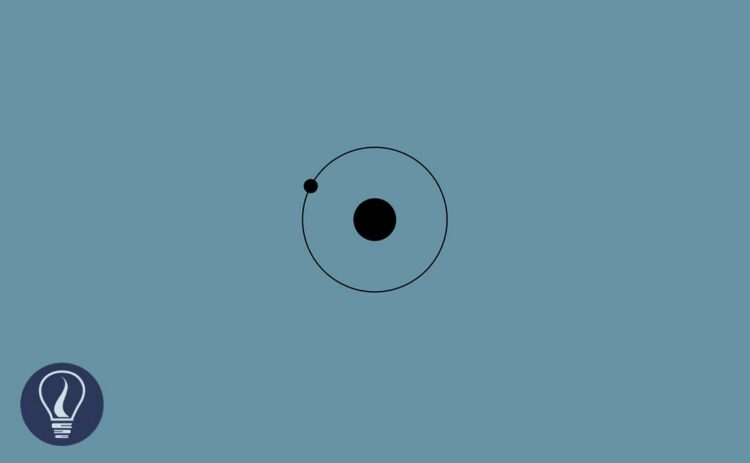More results...

Generic selectors
Exact matches only
Search in title
Search in content
Post Type Selectors

# Mass Calculation of AtomsB.Sc (Hons, USJ) (Polymer Science and Technology, Chemistry, Physics)
Categories
Tags :
Last Updated On : January 20, 2023
Published Date : December 15, 2022

## Mass Calculation of Atoms

Basically, an atom consists of electrons, protons, and neutrons. when compared to the mass of protons and neutrons the mass of electrons is negligible. Therefore, the contribution of the mass comes from neutrons and protons in the nucleus of the atom.

Since these particles have small masses, it is difficult to express their masses in standard mass units (SI units) like Kilograms (Kg) or Grams (g). Therefore, a new unit has been introduced to express the masses of atoms.

## Atomic mass unit (a.m.u.)

The atomic mass unit is defined as the one-twelfth of the C – 12 isotope. C- 12 isotope has six protons and six neutrons in the nucleus. These protons and neutrons are responsible for the mass of the atom. To take an average value, the mass of the nucleus is divided by 12. In other words, the atomic mass unit is the average value of proton rest mass and neutron rest mass.

To calculate the atomic mass unit, the rest mass of protons and neutrons is taken. Rest mass is the mass that shows when the particles are stationary relative to the observer. All the atoms in the matter at any state are in constant motion. And they have high velocity. According to the theory of relativity, the mass is higher when they are moving than they are stationary. To get the minimum mass, rest mass should be taken.

## Relative atomic mass

The ratio of the mass of an atom to the atomic mass unit is known as the relative atomic mass.

### Example 01: Calculating relative atomic mass

If the mass of an atom of a particular element is 6.642×10-26 kg, find the relative atomic mass of the element.

## Relative molecular mass

Generally relative molecular mass is the ratio of the mass of a molecule to the atomic mass unit. But it can be simply found by adding all the relative atomic masses of the atoms in the molecule.

### Example 02: Calculating relative molecular mass

Find the relative molecular mass of CaCO3. Relative atomic masses; Ca - 40.078 u, C - 12.011 u, O - 15.999 u.## References and Attributes

### Figures:

The cover image was created using an image by Nicolás Damián Visceglio from Pixabay